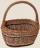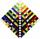# Trees

A certain species of tree grows an average of 0.5 cm per week. Write an equation for the sequence that represents the weekly height of this tree in centimeters if the measurements begin when the tree is 200 centimeters tall.

Result

x(n) = (Correct answer is: 200+0.5(n-1))#### Solution:Leave us a comment of example and its solution (i.e. if it is still somewhat unclear...):

Showing 0 comments:Be the first to comment!## Next similar examples:

1. SequenceWrite the first 7 members of an arithmetic sequence: a1=-3, d=6.
2. Sequence 2Write the first 5 members of an arithmetic sequence a11=-14, d=-1
3. SequenceWrite the first 6 members of these sequence: a1 = 5 a2 = 7 an+2 = an+1 +2 an
4. Sequence 3Write the first 5 members of an arithmetic sequence: a4=-35, a11=-105.
5. Linear systemSolve a set of two equations of two unknowns: 1.5x+1.2y=0.6 0.8x-0.2y=2
6. Two equationsSolve equations (use adding and subtracting of linear equations): -4x+11y=5 6x-11y=-5
7. AP - simpleDetermine the first nine elements of sequence if a10 = -1 and d = 4
8. SeatsSeats in the sport hall are organized so that each subsequent row has five more seats. First has 10 seats. How many seats are: a) in the eighth row b) in the eighteenth row
9. Nineteenth memberFind the nineteenth member of the arithmetic sequence: a1=33 d=5 find a19
10. Theorem proveWe want to prove the sentense: If the natural number n is divisible by six, then n is divisible by three. From what assumption we started?
11. 6 termsFind the first six terms of the sequence. a1 = 7, an = an-1 + 6
12. AS sequenceIn an arithmetic sequence is given the difference d = -3 and a71 = 455. a) Determine the value of a62 b) Determine the sum of 71 members.
13. SequenceBetween numbers 1 and 53 insert n members of the arithmetic sequence that its sum is 702.
14. Apples in basketsDetermine how many apples are in baskets when in the first basket are 4 apples, and in any other is 29 apples more than the previous, and we have eight baskets.
15. SeriesYour task is express the sum of the following arithmetic series for n = 14: S(n) = 11 + 13 + 15 + 17 + ... + 2n+9 + 2n+11
16. One halfOne half of ? is: ?
17. AP - simpleFind the first ten members of the sequence if a11 = 132, d = 3.